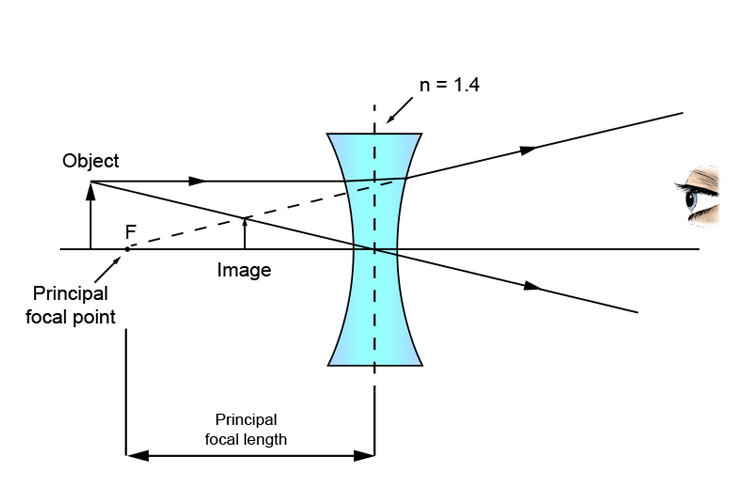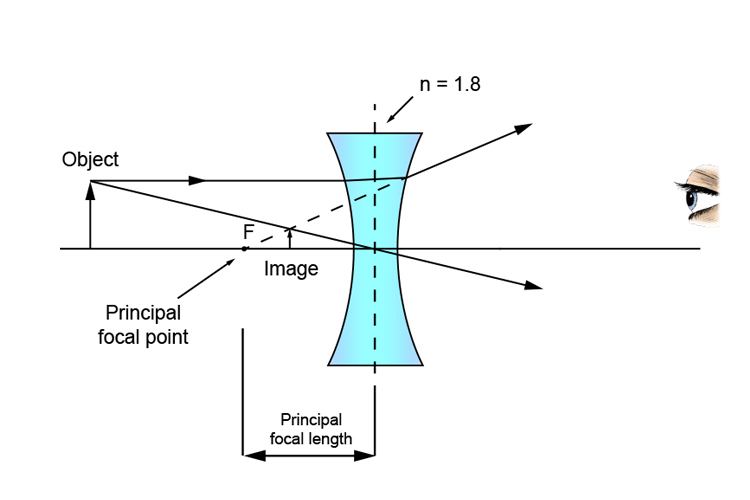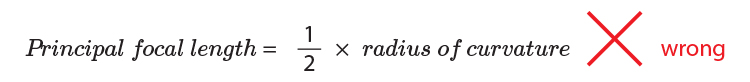# Principal focal point and refractive index

Unlike mirrors, different concave lenses of the same size (i.e. ones with the same centre of curvature which defines how flat or curved the concave lens is) can have different principal focus points.

So much depends on the material the concave lens is made of and the angle of refraction that this creates. You have to know the refractive index of the material (n).

ExampleNOTE: For comparison air n=1   glass n=1.5   diamond n=2.4

Higher refractive index = shorter principal focal point

This makes sense and should be easy to remember because the higher the refractive index, the more the light ray refracts, therefore having a greater effect (see Refractive index section Mammoth Memory).

NOTE: In some books you may read that the principal focal length of a lens isYou can see from the above that this cannot possibly be correct because for lenses of the same curvature, different materials will have a different principal focal length.